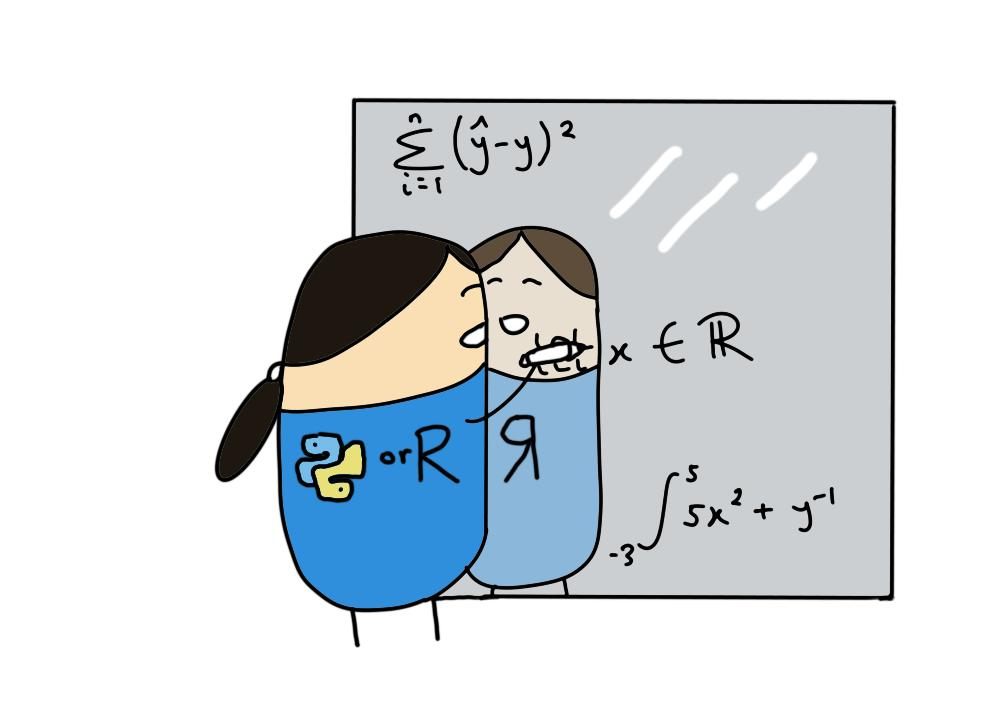# Introductory_maths

A repository of tutorials to revise mathematical concepts required for statistics and machine learning
Alternatives To Introductory_maths
Awesome Math6,830
a month ago8Python
A curated list of awesome mathematics resources
Mathnet Numerics3,0971,3353533 days ago114April 03, 2022273mitC#
Math.NET Numerics
Learn_math_fast2,920
3 years ago3Python
This is the Curriculum for "How to Learn Mathematics Fast" By Siraj Raval on Youtube
Unplugged2,542
2 days ago10TeX
Open book about math and programming.
Math Php2,17036222 months ago132April 10, 202250mitPHP
Powerful modern math library for PHP: Features descriptive statistics and regressions; Continuous and discrete probability distributions; Linear algebra with matrices and vectors, Numerical analysis; special mathematical functions; Algebra
Ml Foundations1,705
5 months agomitJupyter Notebook
Machine Learning Foundations: Linear Algebra, Calculus, Statistics & Computer Science
Hackermath1,339
5 years ago5mitJupyter Notebook
Introduction to Statistics and Basics of Mathematics for Data Science - The Hacker's Way
Awesome Scientific Computing925
3 months agocc0-1.0Python
:sunglasses: Curated list of awesome software for numerical analysis and scientific computing
Egison859
The Egison Programming Language
Algebrite8501710a year ago37April 14, 202179mitTypeScript
Computer Algebra System in Javascript (Typescript)
Alternatives To Introductory_maths
Select To Compare

Alternative Project Comparisons

# Introductory mathematics in R and Python

This repository contains tutorials on the introductory mathematical concepts required for studying statistics and machine learning. Code to solve mathematical problems is written in `R`, `Python` and `Julia`.## Tutorials

Topics Tutorials
🔢 Introduction to numbers (Updated)
🔢 Introduction to algebra
🌗 Introduction to set theory (Updated)
:compass: Introduction to trigonometry
🍪 Introduction to summations
🍪 Introduction to combinatorics (Updated)
🔢 Introduction to functions
🎢 Introduction to derivatives
🎢 Introduction to integration
🎢 Differential equations
🎢 Multivariable functions
🎢 Differentiation of multivariable functions
🔢 Exponents and logarithms
🔢 Logarithms and information theory
🃏 Introduction to probability theory
🃏 Conditional probability
🃏 Bayes theorem
:compass: Introduction to distance metrics
:compass: Cosine similarity applications
:chopsticks: Introduction to linear systems
:chopsticks: Introduction to vectors
:chopsticks: Vector norms and embeddings
🏬 Introduction to matrices
:chopsticks: Linear transformations
:chopsticks: Applications of eigenvalues and eigenvectors

## Project setup

This project was created using the following setup:

• R package dependencies are managed using renv for R version 4.1.2 (2021-11-01).
• Python virtual environment and package dependencies are managed using `poetry` for `Python 3.9.6`. A local version of `Python 3.9.6` was installed and activated using `pyenv local 3.9.6` via the terminal.
• The Julia version used is `julia version 1.7.3`.

## Guide to writing mathematical proofs

Writing mathematical proofs might feel archaic but they are a great way to help you reason why mathematical concepts should behave consistently (and not just because your textbook says so). There are multiple approaches to proving a mathematical statement or concept. Sadly, there is no magical rule to selecting the correct method for each scenario - mathematicians often have to try multiple approaches before they find the right one.

Direct proof

• Occurs when you need to prove that A and B are equivalent.
• Start by assuming A is true.
• Construct a definition statement for A (use a fixed but arbitary example of A).
• Extend and simplify mathematical definitions derived from A to reach B.
• When you are asked if A and only A is true, then B is true, first suppose A to reach B. Then suppose B to reach A.

Induction proof

• Occurs when you need to prove that something is true for all cases.
• Start by proving the base case when \$n = 1\$.
• Assume that the case is also true for some integer \$k\$.
• Prove that the case for \$k + 1\$ also holds i.e. prove the next incremental step up a ladder stretching to infinity.

Uniqueness proof

• Occurs when you need to prove that a solution is unique.
• Show that there is one solution first.
• Show that there is a second solution and that the first and second solutions must be equal.

• Start by assuming that the incorrect state is true i.e. that eigenvectors are linearly dependent.
• Prove that the assumption does not hold and contradicts itself.
• Therefore prove that the reverse state is actually true.

## References

Popular Mathematics Projects
Popular Algebra Projects
Popular Mathematics Categories
Related Searches

Get A Weekly Email With Trending Projects For These Categories
No Spam. Unsubscribe easily at any time.
Python
Html
R
Mathematics
Algebra
Matrices
Calculus
Linear Algebra